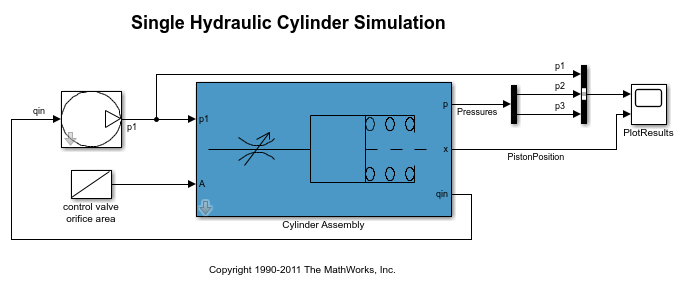# fastRestart

Simulate Simulink model in fast restart mode using simulation scenario

## Syntax

```Simulator_out = fastRestart(Simulator,EnablefastRestart) ```

## Description

`Simulator_out = fastRestart(Simulator,EnablefastRestart)` configures a Simulink® model and simulation scenario specified in `sdo.SimulationTest` object, `Simulator`, for simulation in fast restart mode. Fast restart configures the model to compile once when first simulated. Subsequent model simulations reuse the compiled data, speeding up subsequent simulation runs.

## Input Arguments

expand all

Simulation scenario for Simulink model, specified as an `sdo.SimulationTest` object. A simulation scenario specifies input signals, model parameter and initial state values, and signals to log for a model.

If you want to linearize the model, for example if you have frequency-domain design requirements, specify the linearization logging information in the `SystemLoggingfInfo` property of `Simulator`.

Configuration of model and simulation scenario for fast restart, specified as one of the following values:

• `'on'` — Initializes a Simulink model to simulate in the fast restart mode using simulation scenario specified in `Simulator` object. Fast restart configures the model to compile once when first simulated. Subsequent model simulations reuse the compiled data, speeding up subsequent simulation runs.

Once you have initialized a model in fast restart, you can change only tunable properties of the model.

• `'off'` — Turns off the fast restart mode. Use this option to change nontunable properties of your model.

## Output Arguments

expand all

Simulation scenario configured for fast restart, returned as a `sdo.SimulationTest` object.

## Examples

expand all

Open the model.

```open_system('sdoHydraulicCylinder') ```Create a simulation scenario for the model.

```simulator = sdo.SimulationTest('sdoHydraulicCylinder'); ```

Configure the model and `simulator` for fast restart.

```simulator = fastRestart(simulator,'on'); ```

Simulate the model.

```simulator = sim(simulator); ```

The first simulation in fast restart mode requires the model to compile. Subsequent simulations execute in fast restart mode and reuse the compiled data.

```Ac = sdo.getParameterFromModel('sdoHydraulicCylinder','Ac'); Ac.Value = 0.5; simulator.Parameters = Ac; ```

Simulate the model again.

```simulator = sim(simulator); ```

The model simulates in fast restart mode.

Turn off fast restart mode.

```simulator = fastRestart(simulator,'off'); ```

## Tips

• To optimize, evaluate, or estimate a Simulink model, first create a simulator configured for fast restart (`Simulator_out`). Then use `Simulator_out` as an input to your cost function. If you create a simulator in the cost function, you cannot use fast restart mode.

• When you enable fast restart, you can change only tunable properties of the model.

• To linearize the model, specify the linearization logging information in the `SystemLoggingfInfo` property of `Simulator` before configuring the model for fast restart.

## Version History

Introduced in R2015b